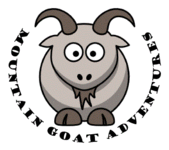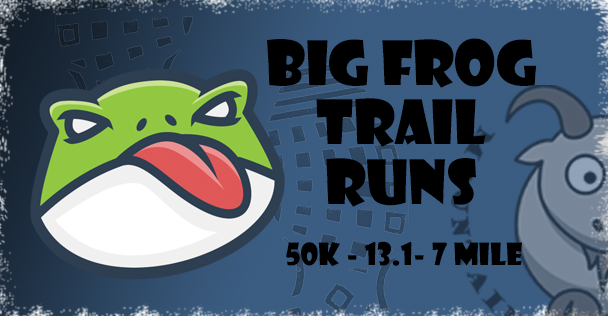# Thank you for signing up for the upcoming Big Frog Trail Runs on April 24th. We hope you all enjoy running these trails as well as relaxing at the race venue after the event. This race update goes over our race day and start procedures for the upcoming event. Knowing these procedures will help us ensure we have a safe and smooth event for everyone. We look forward to seeing everyone on April 24th!

Please start by clicking on the 2 big green buttons below to:
1. Verify your details
2. Print the race accident waiver

## Address of Ocoee Whitewater Center (RACE START): 4400 US-64, Copperhill, TN 37317

The race begins and ends at the Ocoee Whitewater Center. To enter the venue in the morning, you will need to actually drive past the Whitewater Center on US 64 and enter from the far end of the parking lot. It is \$3 per vehicle to park for the day, which can be paid at the USFS kiosks when you arrive. Please bring exact change for the fee envelopes in the “iron ranger”. Once you park, walk across the bridge and make a left towards the picnic shelter, which is where check-in will be. PLEASE NOTE THERE IS NO OVERNIGHT PARKING AT THE OCOEE WHITEWATER CENTER – SEE EVENT WEBSITE FOR CAMPING OPTIONS.

## Race Check-In: (2 Options)

Runners may check in on Saturday April 23 from 4 pm to 8 pm, or on Sunday April 24 from 6 am to 7:30 am. Race check-in will be at the Pavilion. You may have someone else check in for you and collect your race bib and swag ONLY if you send a signed waiver with them. You may have another racer pick up your swag for you if are unable to attend the race.

## Schedule:

7:40 am - Pre-race meeting for the Big Frog 50K near the pavilion
8:00 am - Big Frog 50K Race Start in Whitewater Center Parking Lot
8:10 am - Pre-race meeting for the 13.1 / 7 mile races near the pavilion
8:30 am - Big Frog 13.1 Start near pavilion
8:40 am - Big Frog 7 Mile Start near pavilion

Awards for each race will be presented near the finish line when the top 3 finishers in each category have crossed the finish line.

## Race Distance Changes:

If you want to switch your race distance, please do so by contacting me no later than Monday April 18th and we will get you taken care of.

## Courses and Aid Stations:

Please see the RACE COURSE MAPS page for these details.

## Chip Timing and Real Time Results:

Your race number has a disposable timing chip adhered to the back of it. Please do not bend or fold this chip. Numbers need to be pinned on your front, preferably on your chest. If you wear a number belt, please ensure the number is on your front when you cross the finish line so your chip reads. Your race time and category placing will be visible almost immediately upon finishing on tablets that will be located near the finish line. Please check your finish time/category for accuracy when you finish and report any discrepancies BEFORE you leave, as results will become official 15 minutes after the last person finishes.

## Miscellaneous Details:

• Course will be well marked with pink arrow signs at turns and pink flagging tape. We use A LOT of course markings and have an extremely low incidence of runners making wrong turns at our events.
* Please print race ACCIDENT WAIVER at home, sign and bring to race check-in with you.
• 50k runners are welcome to have support crews, however they may only meet and assist runners at aid stations and must not interfere with other runners on course. Crews may need to park a distance away from the aid stations and walk as parking is limited in some areas.
• Crew vehicles cannot block ANY roads and crews may not interfere with other runners on course or at aid stations.
• No pacers or other unregistered runners on course unless running with a minor
• 50k runners may send a "drop bag" that is one gallon-ish size to Boyd Gap (Mile 9) and Chestnut Mountain/FS 221 (Mile 20.3 and Mile 27.6). These need to be turned in at registration by 7:45 am and we will transport to the aid stations for you. Write your number BIG on the bag for faster retrieval by our volunteers.
• Poles are allowed if you want to use them
• Dogs are allowed at the Ocoee Whitewater Center but they must be on a 6’ leash and you must be prepared to clean up after them
• Post-Race snacks, soda and water will be available for 7 and 13.1 mile runners.
• A post-race lunch with soda or water will be available for 50k runners as they finish.
* If you can no longer attend, you may sell your entry to someone else, but this needs to be done by 4/18. Payment is between buyer and seller..all I need is the new runner's name and email no later than 4/18. Sorry, no late transfers, and no transfers to other events at this point.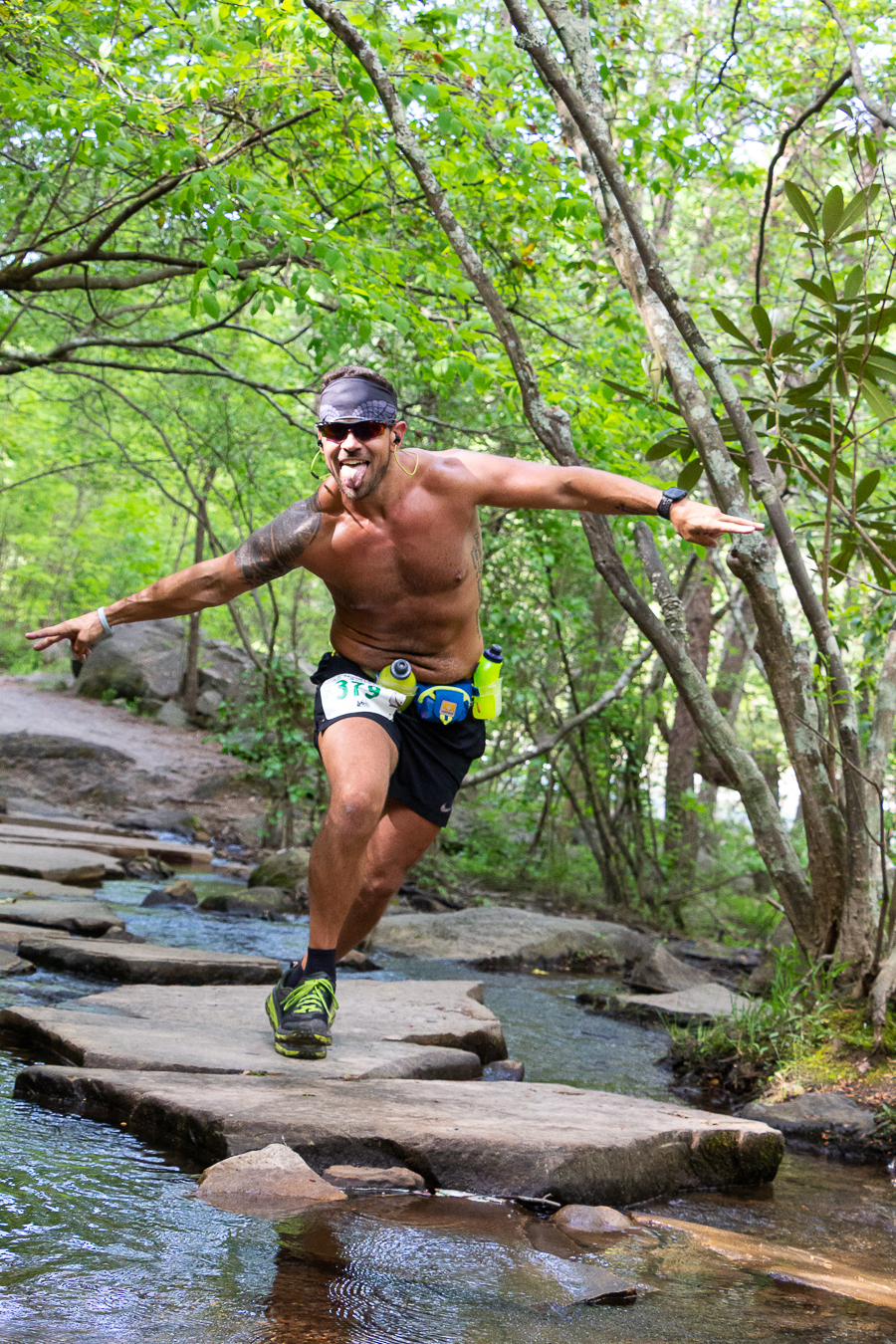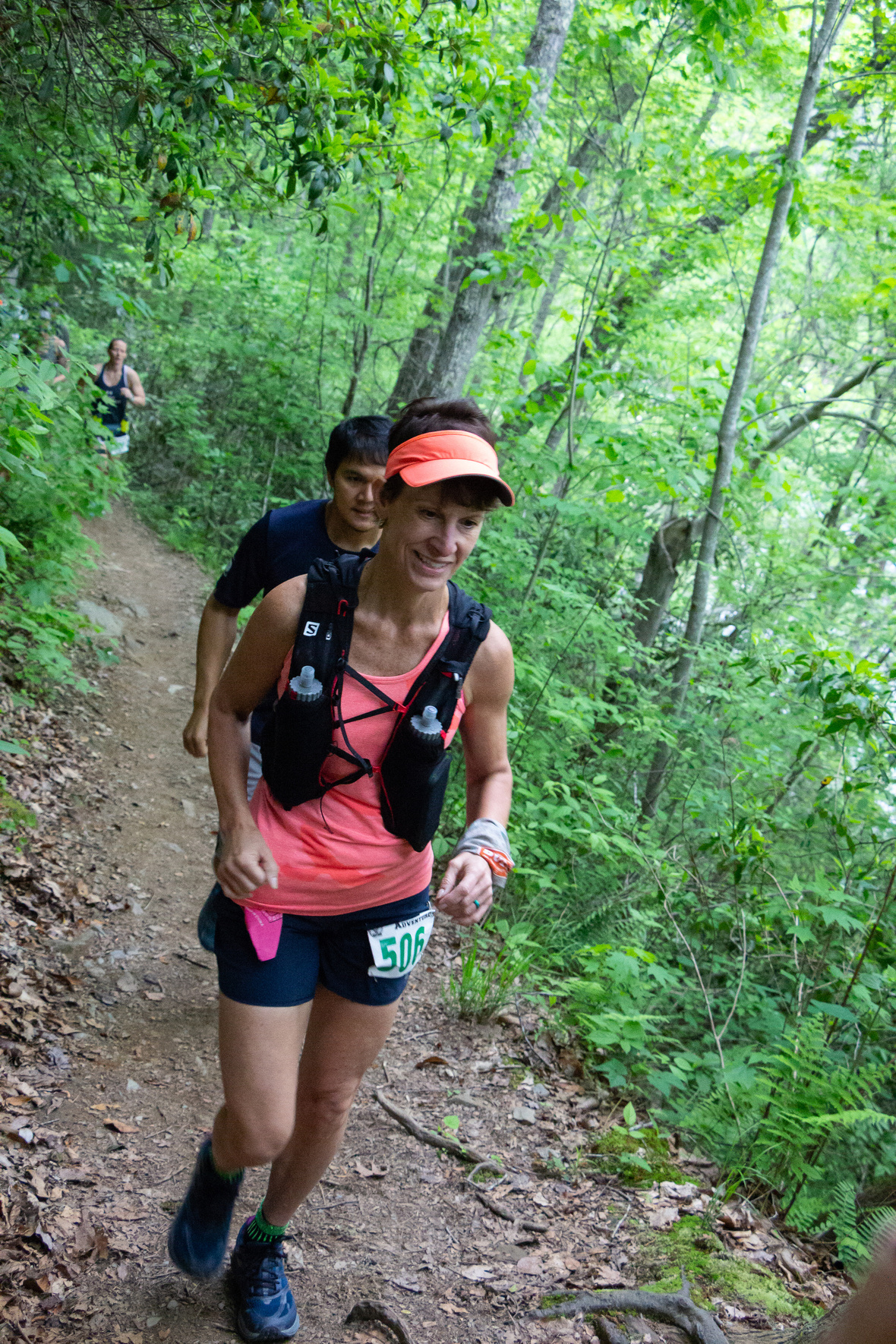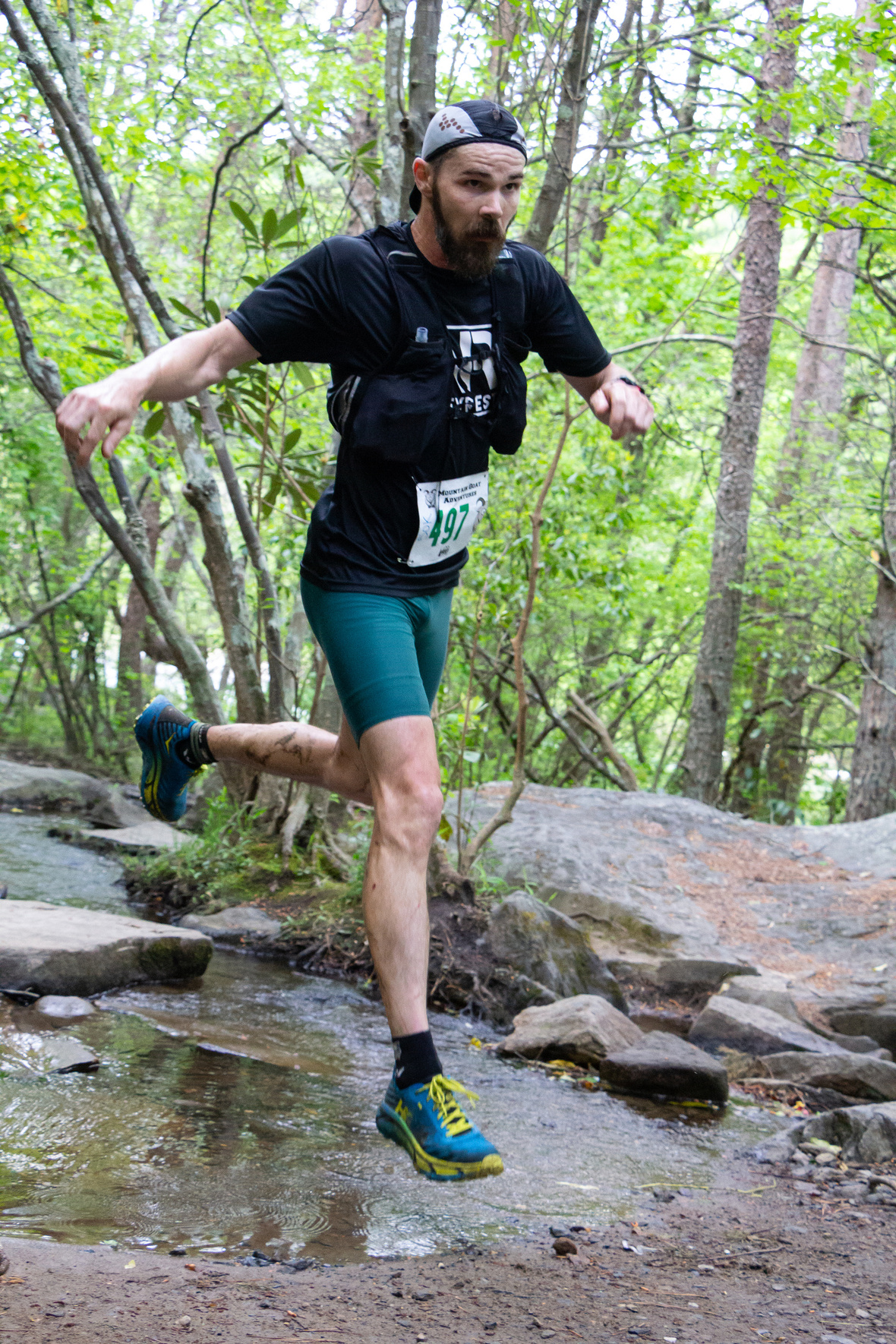table div table+table+table+table+table+table+table+table div table{width:100%;padding:0}table div table+table+table+table+table+table+table+table div table img{width:96.23%;padding:0;float:none}table div table+table+table+table+table+table+table+table div table td{width:100%;padding:0 1.88% 18px}/* styles */## Hammer Nutrition Race Day Fueling Tips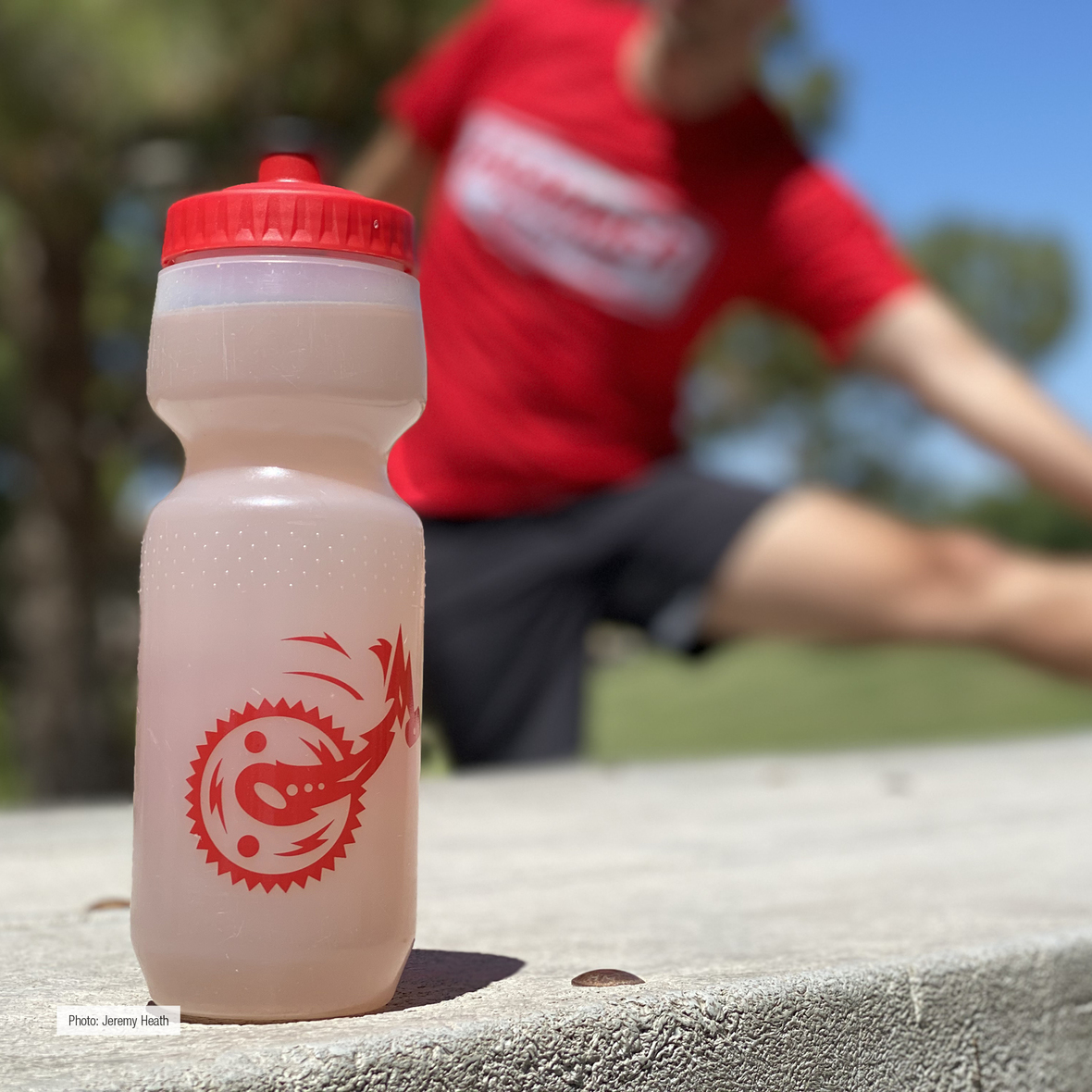Hammer Nutrition endurance fuels will be on the course at the 2022 Big Frog Trail Runs!

Hammer’s been supplying athletes with natural products free of added sugars, artificial colors, flavors, chemicals, and preservatives for over 30 years.

Your body needs to run at peak capacity so you can get your best result during the event. Do you have your nutrition, hydration, and fueling needs locked down? Follow these 5 tips to avoid sending an SOS during the event:

Tip #1 Hydration: Replenish, don’t replace! Keep fluid intake below 25 oz./hr during exercise. FIND OUT HOW MUCH

Tip #2 Caloric Intake: Stay fueled, but not overwhelmed, by consuming 120-180 calories/hr. ARE CARBS BEST?

Tip #3 Electrolytes: You need an array of vitamins and minerals, not just salt, to finish strong. WHAT DO YOU NEED?

Tip #4 Pre-Event Fueling: Don’t tax your system with a meal within 3 hours of start time. BUT WHAT IF YOU'RE HUNGRY?

Tip #5 Recovery: Refuel your tank with 60 minutes of finishing a workout. ARE CARBS OR PROTEINS BETTER?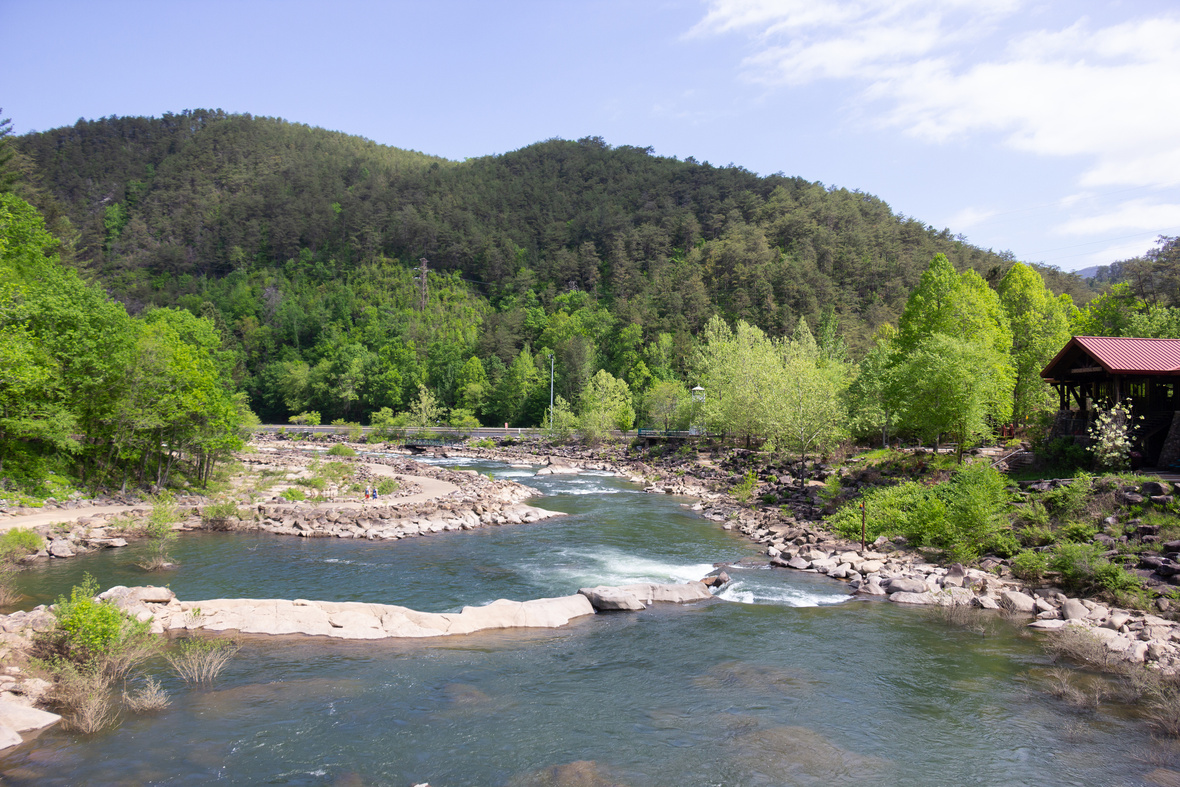table div table+table+table+table+table+table+table+table+table+table+table+table div table{width:100%;padding:0}table div table+table+table+table+table+table+table+table+table+table+table+table div table img{width:96.23%;padding:0;float:none}table div table+table+table+table+table+table+table+table+table+table+table+table div table td{width:100%;padding:0 1.88% 18px}/* styles */table div table+table+table+table+table+table+table+table+table+table+table+table+table div table{width:100%;padding:0}table div table+table+table+table+table+table+table+table+table+table+table+table+table div table img{width:96.23%;padding:0;float:none}table div table+table+table+table+table+table+table+table+table+table+table+table+table div table td{width:100%;padding:0 1.88% 18px}/* styles */## 2022 Full Event Calendar

 /* styles */ Want to check out our other events? Our 2022 calendar is below - we focus on mountain bike races, trail runs, and off-road duathlons. April 23, 2022 - Old Copper 30/Big Frog 65/Cohutta 100 MTB Races April 24, 2022 - Big Frog 50k - 13.1 mile - 7 mile Trail Runs May 14, 2022 - Assault on Garland Mountain 10k and 13.1 mile trail run September 10, 2022 - Fools Gold 38 and 60 Mile MTB Races October 1, 2022 - Blankets Creek 3/6 Hour Race November 12, 2022 - Rope Mill Dirty Duathlon and 5k Trail Run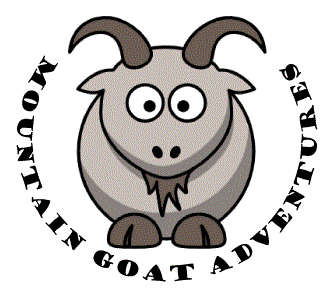## Thanks to our Trail Run Sponsors!

 table div table+table+table+table+table+table+table+table+table+table+table+table+table+table+table+table+table+table div table td,table.module-17{width:100%;padding:0}table div table+table+table+table+table+table+table+table+table+table+table+table+table+table+table+table+table+table div table{width:100%;float:none;margin-left:auto;margin-right:auto;padding:0}table div table+table+table+table+table+table+table+table+table+table+table+table+table+table+table+table+table+table div table a{border:0 none;text-decoration:none}table div table+table+table+table+table+table+table+table+table+table+table+table+table+table+table+table+table+table div table img{width:100%!important;border:0 none;text-decoration:none}/* styles */
 table.module-18{width:84.72%;padding:0}table div table+table+table+table+table+table+table+table+table+table+table+table+table+table+table+table+table+table+table div table{width:84.72%;float:none;margin-left:auto;margin-right:auto;padding:0}table div table+table+table+table+table+table+table+table+table+table+table+table+table+table+table+table+table+table+table div table a{border:0 none;text-decoration:none}table div table+table+table+table+table+table+table+table+table+table+table+table+table+table+table+table+table+table+table div table img{width:100%!important;border:0 none;text-decoration:none}table div table+table+table+table+table+table+table+table+table+table+table+table+table+table+table+table+table+table+table div table td{width:100%;padding:0}/* styles */
 table.module-19{width:75.66%;padding:0}table div table+table+table+table+table+table+table+table+table+table+table+table+table+table+table+table+table+table+table+table div table{width:75.66%;float:none;margin-left:auto;margin-right:auto;padding:0}table div table+table+table+table+table+table+table+table+table+table+table+table+table+table+table+table+table+table+table+table div table a{border:0 none;text-decoration:none}table div table+table+table+table+table+table+table+table+table+table+table+table+table+table+table+table+table+table+table+table div table img{width:100%!important;border:0 none;text-decoration:none}table div table+table+table+table+table+table+table+table+table+table+table+table+table+table+table+table+table+table+table+table div table td{width:100%;padding:0}/* styles */
 table div table+table+table+table+table+table+table+table+table+table+table+table+table+table+table+table+table+table+table+table+table div table{width:100%;padding:0}table div table+table+table+table+table+table+table+table+table+table+table+table+table+table+table+table+table+table+table+table+table div table table{padding:0;float:left!important;width:61.32%!important}table div table+table+table+table+table+table+table+table+table+table+table+table+table+table+table+table+table+table+table+table+table div table table+table td,table div table+table+table+table+table+table+table+table+table+table+table+table+table+table+table+table+table+table+table+table+table div table td{padding-left:0;padding-right:0}table div table+table+table+table+table+table+table+table+table+table+table+table+table+table+table+table+table+table+table+table+table div table table td{padding-left:0;padding-right:20px}table div table+table+table+table+table+table+table+table+table+table+table+table+table+table+table+table+table+table+table+table+table div table table+table{float:left!important;width:38.68%!important}/* styles */
 table div table+table+table+table+table+table+table+table+table+table+table+table+table+table+table+table+table+table+table+table+table+table div table{width:100%;padding:0}table div table+table+table+table+table+table+table+table+table+table+table+table+table+table+table+table+table+table+table+table+table+table div table table{padding:0;float:left!important;width:56.415%!important}table div table+table+table+table+table+table+table+table+table+table+table+table+table+table+table+table+table+table+table+table+table+table div table table+table td,table div table+table+table+table+table+table+table+table+table+table+table+table+table+table+table+table+table+table+table+table+table+table div table td{padding-left:0;padding-right:0}table div table+table+table+table+table+table+table+table+table+table+table+table+table+table+table+table+table+table+table+table+table+table div table table td{padding-left:0;padding-right:20px}table div table+table+table+table+table+table+table+table+table+table+table+table+table+table+table+table+table+table+table+table+table+table div table table+table{float:left!important;width:43.585%!important}/* styles */
 table.module-22{width:50.38%;padding:0}table div table+table+table+table+table+table+table+table+table+table+table+table+table+table+table+table+table+table+table+table+table+table+table div table{width:50.38%;float:none;margin-left:auto;margin-right:auto;padding:0}table div table+table+table+table+table+table+table+table+table+table+table+table+table+table+table+table+table+table+table+table+table+table+table div table a{border:0 none;text-decoration:none}table div table+table+table+table+table+table+table+table+table+table+table+table+table+table+table+table+table+table+table+table+table+table+table div table img{width:100%!important;border:0 none;text-decoration:none}table div table+table+table+table+table+table+table+table+table+table+table+table+table+table+table+table+table+table+table+table+table+table+table div table td{width:100%;padding:0}/* styles */
 table div table+table+table+table+table+table+table+table+table+table+table+table+table+table+table+table+table+table+table+table+table+table+table+table div table{width:100%;padding:0}table div table+table+table+table+table+table+table+table+table+table+table+table+table+table+table+table+table+table+table+table+table+table+table+table div table table{padding:0;float:left!important;width:59.811%!important}table div table+table+table+table+table+table+table+table+table+table+table+table+table+table+table+table+table+table+table+table+table+table+table+table div table table+table td,table div table+table+table+table+table+table+table+table+table+table+table+table+table+table+table+table+table+table+table+table+table+table+table+table div table td{padding-left:0;padding-right:0}table div table+table+table+table+table+table+table+table+table+table+table+table+table+table+table+table+table+table+table+table+table+table+table+table div table table td{padding-left:0;padding-right:20px}table div table+table+table+table+table+table+table+table+table+table+table+table+table+table+table+table+table+table+table+table+table+table+table+table div table table+table{float:left!important;width:40.189%!important}/* styles */
 table.module-24{width:54.34%;padding:0}table div table+table+table+table+table+table+table+table+table+table+table+table+table+table+table+table+table+table+table+table+table+table+table+table+table div table{width:54.34%;float:none;margin-left:auto;margin-right:auto;padding:0}table div table+table+table+table+table+table+table+table+table+table+table+table+table+table+table+table+table+table+table+table+table+table+table+table+table div table a{border:0 none;text-decoration:none}table div table+table+table+table+table+table+table+table+table+table+table+table+table+table+table+table+table+table+table+table+table+table+table+table+table div table img{width:100%!important;border:0 none;text-decoration:none}table div table+table+table+table+table+table+table+table+table+table+table+table+table+table+table+table+table+table+table+table+table+table+table+table+table div table td{width:100%;padding:0}/* styles */